﻿ Mathematical Examples In The Notebook, Calculations Stock Photo - Image of algebra, school: 144011694

Mathematical examples in the notebook, calculations.

Mathematical examples in the notebook, calculations.

Photo Taken On: June 05th, 2017

More similar stock images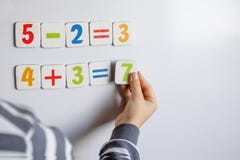The boy solves mathematical examples. A child solves examples on a blackboardSchool supplies on table in white background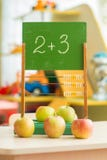Vintage wooden abacus on the tableA board with mathematical examples and a teacher at the table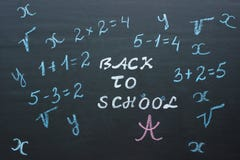Text Back to school and mathematical examples on black chalkboar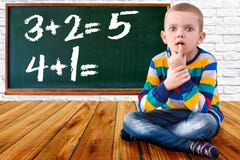Baby boy solve mathematics examples.The student thinks and feels near the Board.Mathematical tasks .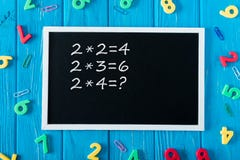top view of blackboard with multiplication table colorful numbers and paper clips on blue wooden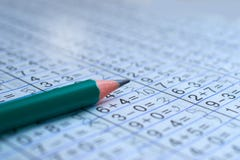Pencil is on notebook with mathematical examples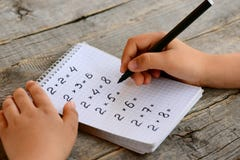Student solves multiplication examples. Child holds a black marker in his hand and writes answers
More stock photos from Andrii Zastrozhnov's portfolio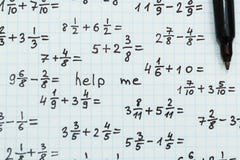Math examples, the inscription - help me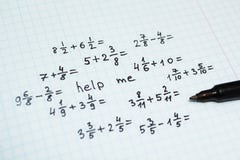Math examples in the notebook in the cage, the inscription - help me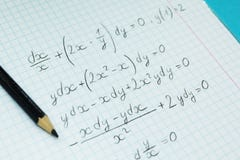Mathematical examples and calculations in a notebook for lectures. The study of arithmetic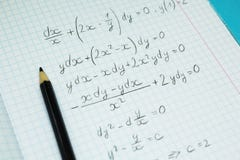Mathematical examples and calculations in a notebook for lectures. Science and education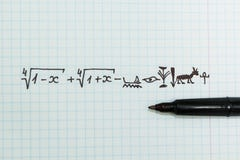Complex mathematical examples in the notebook as Egyptian hieroglyphs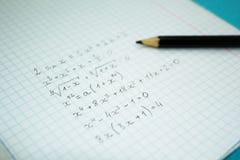Mathematical examples and calculations in a notebook for lectures
Related categories